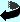# Equilibrium calculation

Insert your data in this form and klick on the "calculate" botton to calculate the equilibrium calculation on basis of the alkalinity conservation approach. The description of this method (Luff, Haeckel and Wallmann) is submitted to Computers & Geosciences. For further details, questions or comments sent an E-Mail to (see below).

If no value for TBOH4 is given (0.0), the total borate concentration will be calculated from the given salinity concentration.

Input values:

 Parameter Unit Range Input Temperature °C 0-40 Salinity PSU (ppt) 0.5-43 Pressure atm (1 atm at surface) 0-1200 Total Alkalinity meq/l TCO2 mmol/l TH2S mmol/l TBOH4 mmol/l

Results:

 Density of bottom water kg/m^3 Concentration of CO2 mmol/l Concentration of HCO3- mmol/l Concentration of CO32- mmol/l Concentration of HS mmol/l Concentration of H2S mmol/l Concentration of T(BOH)4 mmol/l Concentration of BOH3 mmol/l Concentration of BOH4- mmol/l pH bottom water Number of iterations Number of new setup attampts*

* setup attampts: if the first choice of the concentration in thermodynamic equilibrium for the Newton-Raphson Method is too bad for the solution, a new calculation with new randomly generated guess values will be started.

Calculated thermodynamic "constants" with dependency of given temperature, salinity and pressure:

 dissociation constant of H2O 1st dissociation constant of CO2(aq) 2nd dissociation constant of CO2(aq) dissociation constant of B(OH)3 1st dissociation constant of H3PO4 2nd dissociation constant of H3PO4 3rd dissociation constant of H3PO4 dissociation constant of HNO3 dissociation constant of NH4 1st dissociation constant of H2S solubility product of CaCO3 (Aragonite) solubility product of CaCO3 (Calcite)

Back